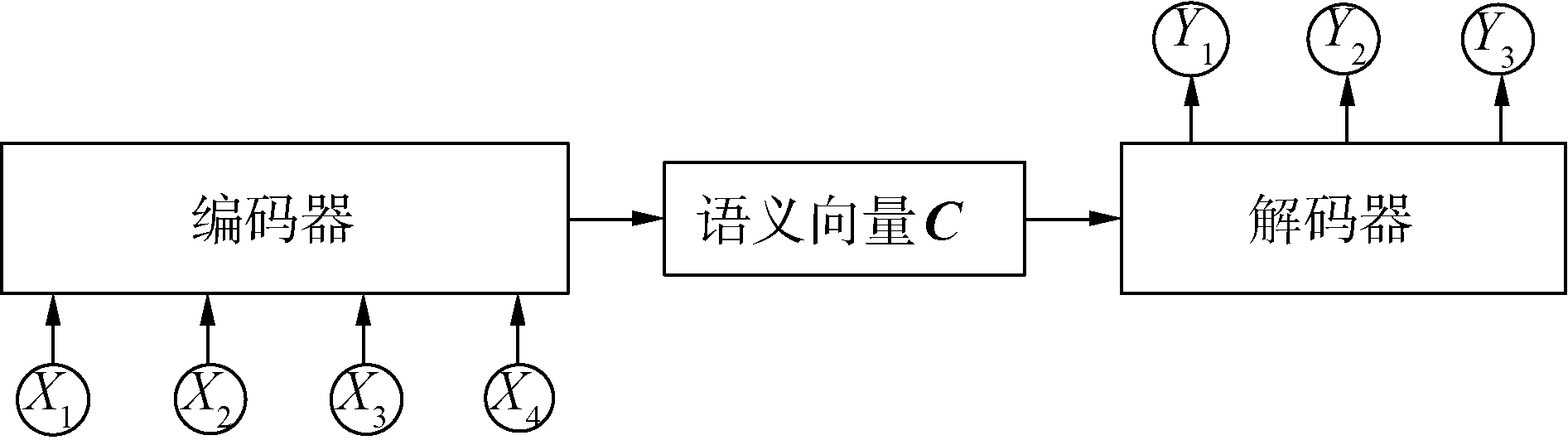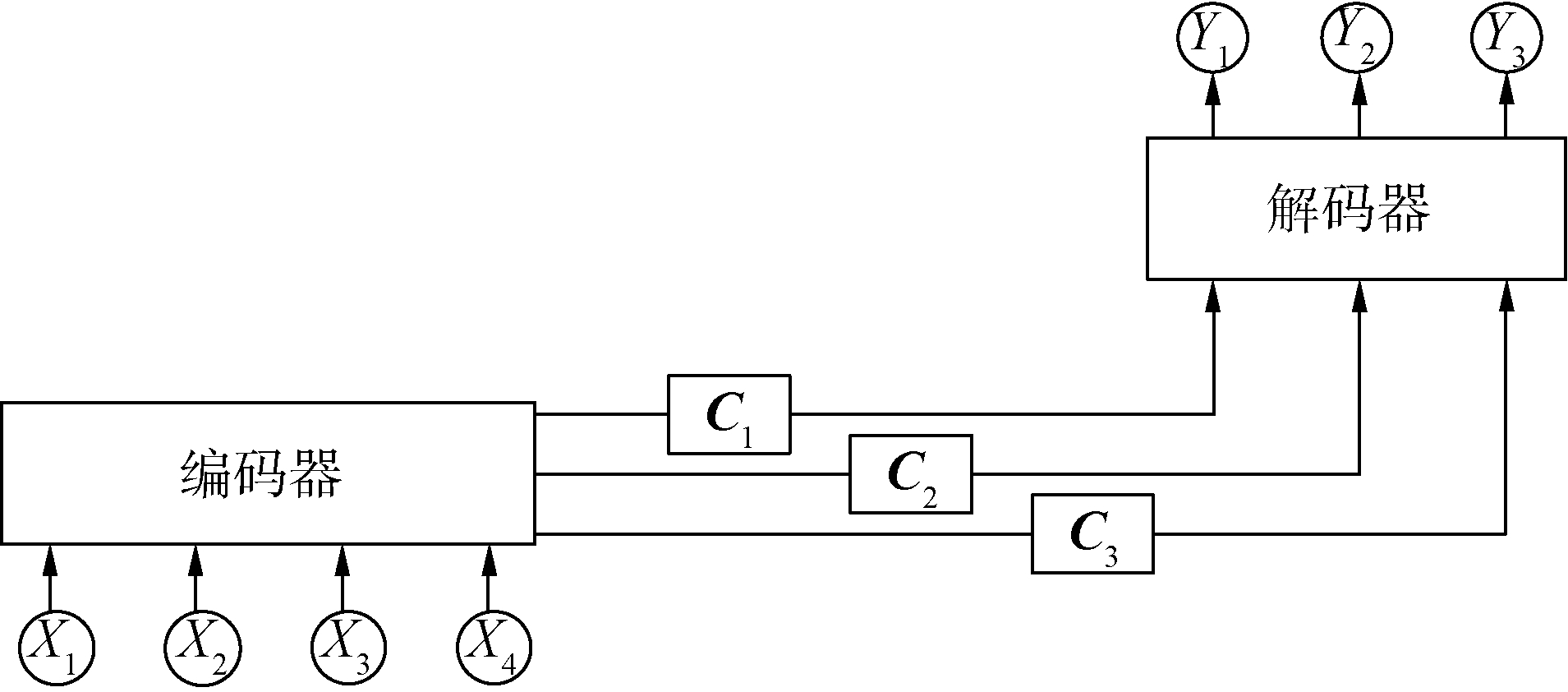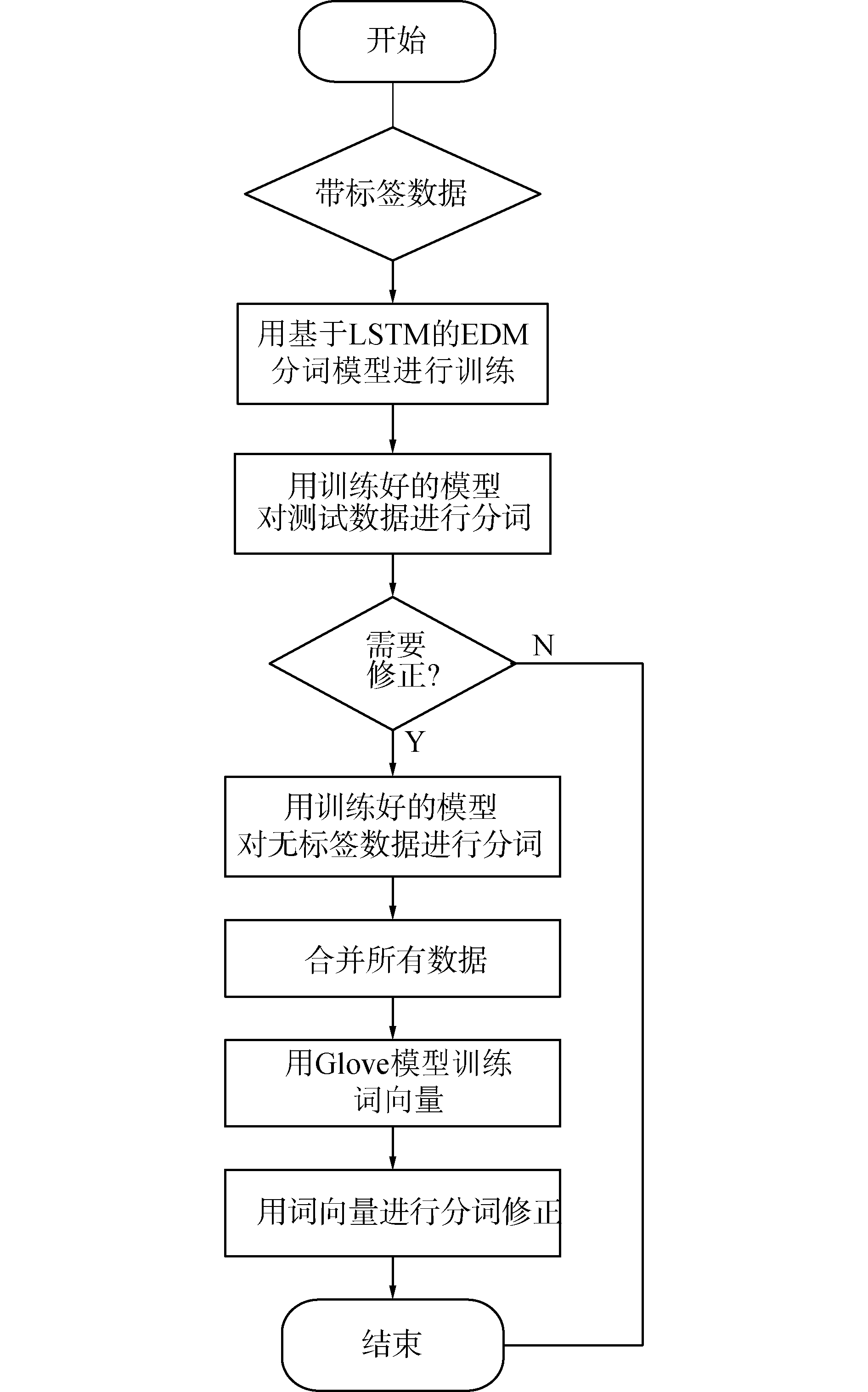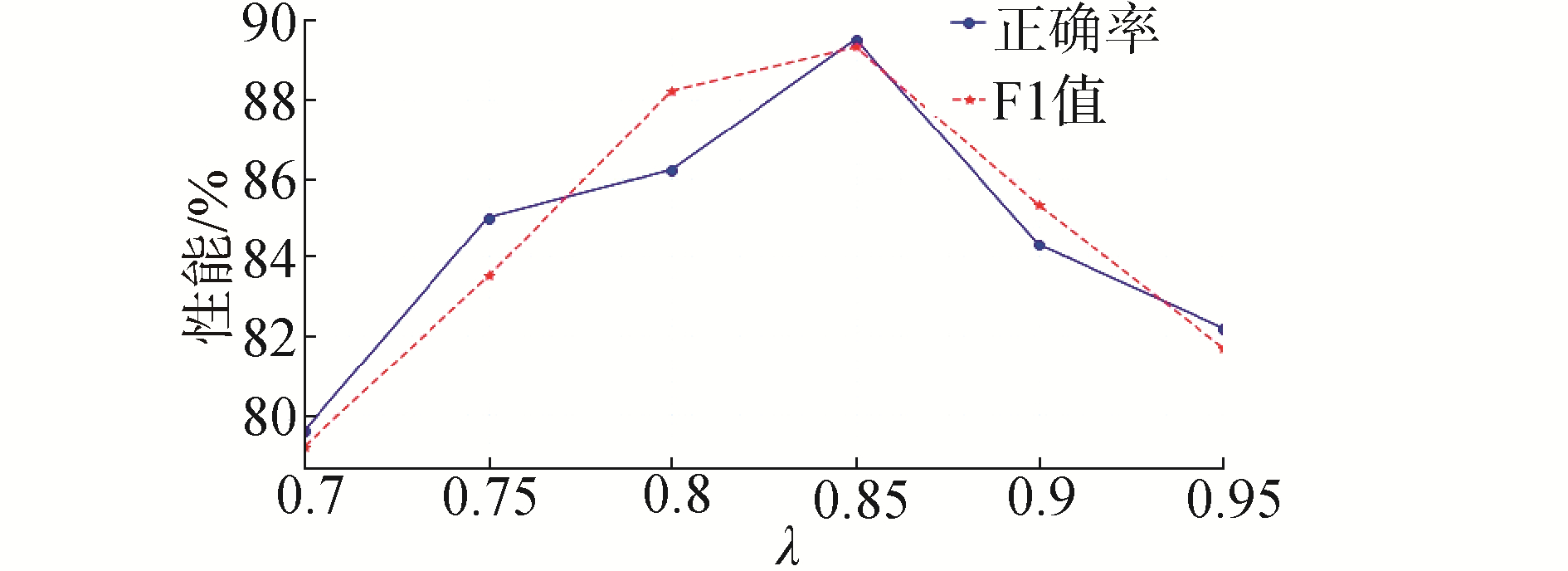﻿ 基于深度神经网络模型的中文分词方案
«上一篇文章快速检索 高级检索

 哈尔滨工程大学学报2019, Vol. 40Issue (9): 1662-1666  DOI: 10.11990/jheu.2018120730

### 引用本文XU Feng, ZHANG Xuefen, XIN Zhanhong. A Chinese word segmentation scheme based on a deep neural network model[J]. Journal of Harbin Engineering University, 2019, 40(9), 1662-1666. DOI: 10.11990/jheu.201812073.### 文章历史

1. 北京邮电大学 经济管理学院, 北京 100876;
2. 北京联合大学 智慧城市学院, 北京 100101

A Chinese word segmentation scheme based on a deep neural network model
XU Feng 1, ZHANG Xuefen 2, XIN Zhanhong 1
1. School of Economics and Management, Beijing University of Posts and Telecommunications, Beijing 100876, China;
2. Smart City College, Beijing Union University, Beijing 100101, China
Abstract: In order to solve the problem of reduced performance of existing segmentation algorithms and programs when processing massive network text segmentation, a Chinese word segmentation scheme based on a deep neural network model is proposed in this paper. The encoder-decoder model (EDM), based on the long short-term memory (LSTM) network, was employed to train the data model, which derived a model to perform the word segmentation. In order to improve the word segmentation performance, a modification method based on word vectors is further provided. Experimental results on the typical Weibo dataset suggested that the performance of the proposed scheme is significantly improved compared with traditional word segmentation software. In addition, word segmentation precision and F-values, after modification by the presented method based on the word vectors, were slightly improved compared with those without modification. All these facts indicated the effectiveness of the proposed segmentation scheme.
Keywords: Chinese word segmentation    long short-term memory network    encoder-decoder model    word vector    accuracy rate    F value

1 基于LSTM的EDM模型 1.1 LSTM模型

LSTM是为了解决传统的RNN模型的梯度消失问题而提出的一种深度神经网络模型。相对于传统的RNN结构，LSTM网络引入记忆单元C和3个逻辑门：输入门(i)、遗忘门(f)和输出门(o)，用来控制信息的传递和遗弃，从而更好地处理历史信息。

1.2 基于LSTM的EDMDownload: 图 1 编码-解码模型 Fig. 1 Encoder-decoder model

 $\mathit{\boldsymbol{C}} = f\left( {{X_1}, {X_2}, \cdots , {X_m}} \right)$ (1)

 ${Y_t} = g\left( {{Y_1}, {Y_2}, \cdots , {Y_{t - 1}}} \right)$ (2)Download: 图 2 引入注意力的编码-解码模型 Fig. 2 Attention-based encoder-decoder model

 ${e_{ij}} = {\mathop{\rm score}\nolimits} \left( {{s_{i - 1}}, {h_j}} \right)$ (3)
 ${\alpha _{ij}} = \frac{{\exp \left( {{e_{ij}}} \right)}}{{\sum\limits_k {\exp } \left( {{e_{ik}}} \right)}}$ (4)
 ${C_i} = \sum\limits_j {{\alpha _{ij}}} {h_j}$ (5)
 ${s_i} = f\left( {{s_{i - 1}}, {y_1}, \cdots , {y_{i - 1}}, {C_i}} \right)$ (6)
 ${y_i} = g\left( {{y_1}, \cdots , {y_{i - 1}}, {s_i}, {C_i}} \right)$ (7)

2 词向量模型

 ${\mathit{\boldsymbol{X}}_i} = \sum\limits_j {{X_{ij}}}$ (8)

 $J(\boldsymbol{W})=\sum\limits_{i, j} f\left(X_{i j}\right)\left(\boldsymbol{W}_{i j}^{\mathrm{T}}-\operatorname{lb}_{2} X_{i j}\right)^{2}$ (9)

 $f\left( {{X_{ij}}} \right) = \left\{ {\begin{array}{*{20}{l}} {{{\left( {{X_{ij}}/{X_{\max }}} \right)}^\alpha }, }&{{X_{ij}} < {X_{\max }}}\\ {1, }&{{X_{ij}} \ge {X_{\max }}} \end{array}} \right.$ (10)

3 分词方案设计与实现 3.1 中文分词方案Download: 图 3 提出的中文分词方案流程图 Fig. 3 The diagram of the proposed scheme for Chinese word segmentation

 $\cos \theta=\frac{<\boldsymbol{W}_{i}, \boldsymbol{W}_{j}>}{\left|\boldsymbol{W}_{i}\right|\left|\boldsymbol{W}_{j}\right|}$ (11)

 ${\mathit{\boldsymbol{W}}_{{\rm{out}}}} = \frac{{{\mathit{\boldsymbol{W}}_i} + {\mathit{\boldsymbol{W}}_{i + 1}}}}{{\left| {{\mathit{\boldsymbol{W}}_i} + {\mathit{\boldsymbol{W}}_{i + 1}}} \right|}}$ (12)

3.2 测试及分析

 $P = \frac{{{D_1}}}{{{D_0}}} \times 100\%$ (13)
 $P = \frac{{{D_1}}}{D} \times 100\%$ (14)
 $F = \frac{{P \times R}}{{(1 - a) \times P + a \times R}}$ (15)Download: 图 4 不同阈值λ下的修正性能 Fig. 4 Correct performances of different threshold λ表 1 不同中文分词方案的性能比较 Table 1 Performance comparisons of different Chinese word segmentation schemes表 2 分词结果示例 Table 2 Examples of the segmentation results
4 结论

1) 提出基于LSTM的EDM分词模型的性能相对于传统的jieba分词软件的分词性能有了较大提升。

2) 采用提出的词向量修正方法修正后的分词准确率和F值略优于未修正的分词准确率。验证了提出的基于LSTM模型的中文分词方案和基于词向量的修正方法的有效性。

  罗刚, 张子宪. 自然语言处理原理与技术实现[M]. 北京: 电子工业出版社, 2016. (0)  黄昌宁, 赵海. 中文分词十年回顾[J]. 中文信息学报, 2007, 21(3): 8-19. HUANG Changning, ZHAO Hai. Chinese Word Segmentation:a decade review[J]. Journal of Chinese information processing, 2007, 21(3): 8-19. DOI:10.3969/j.issn.1003-0077.2007.03.002 (0)  黄昌宁. 中文信息处理中的分词问题[J]. 语言文字应用, 1997(1): 72-78. (0)  WU Andi, JIANG Zixin. Word segmentation in sentence analysis[C]//Proceedings of the 1998 International Conference on Chinese Information Processing. Beijing, 1998: 169-180. (0)  UTIYAMA M, ISAHARA H. A statistical model for domain-independent text segmentation[C]//Proceedings of the 39th Annual Meeting on Association for Computational Linguistics. Toulouse, France, 2001: 499-506. (0)  LOW J K, NG H T, GUO Wenyuan. A maximum entropy approach to Chinese word segmentation[C]//Proceedings of the 4th Sighan Workshop on Chinese Language Processing. Jeju Island, Korea, 2005: 161-164. (0)  ZHAO Hai, HUANG Changning, LI Mu. An improved Chinese word segmentation system with conditional random field[C]//Proceedings of the 5th SIGHAN Workshop on Chinese Language Processing. Sydney, 2006: 162-165. (0)  XUE Nianwen. Chinese word segmentation as character tagging[J]. Computational linguistics and Chinese language processing, 2003, 8(1): 29-48. (0)  TSENG H, CHANG Pichuan, ANDREW G, et al. A conditional random field word segmenter for Sighan bakeoff 2005[C]//Proceedings of the 4th SIGHAN Workshop on Chinese Language Processing, Association for Computational Linguistics. 2005: 168-171. (0)  CHANG Pichuan, GALLEY M, MANNING C D. Optimizing Chinese word segmentation for machine translation performance[C]//Proceedings of the 3rd Workshop on Statistical Machine Translation. Columbus, Ohio, 2008: 224-232. (0)  刘颖.网络语言的变异分析: 现象、成因及发展趋势[D].福州: 福建师范大学, 2012. LIU Ying. Linguistic variation of netspeak: phenomenon, reasons and future developments[D]. Fuzhou: Fujian Normal University, 2012. (0)  HINTON G E, SALAKHUTDINOV R R. Reducing the dimensionality of data with neural networks[J]. Science, 2006, 313(5786): 504-507. DOI:10.1126/science.1127647 (0)  HOCHREITER S, SCHMIDHUBER J. Long short-term memory[J]. Neural computation, 1997, 9(8): 1735-1780. DOI:10.1162/neco.1997.9.8.1735 (0)  CHO K, VAN MERRIENBOER B, GÜLÇEHRE Ç, et al. Learning phrase representations using RNN encoder-decoder for statistical machine translation[C]//Proceedings of the 2014 Conference on Empirical Methods in Natural Language Processing. Doha, Qatar, 2014: 1724-1734. (0)  BAHDANAU D, CHO K, BENGIO Y. Neural machine translation by jointly learning to align and translate[C]//Proceedings of 2015 International Conference on Learning Representations. 2015: 1-15. (0)  LAI Siwei, LIU Kang, HE Shi, et al. How to generate a good word embedding?[J]. IEEE intelligent systems, 2016, 31(6): 5-14. DOI:10.1109/MIS.2016.45 (0)  沈翔翔, 李小勇. 使用无监督学习改进中文分词[J]. 小型微型计算机系统, 2017, 38(4): 744-748. SHEN Xiangxiang, LI Xiaoyong. Improving Chinese word segmentation via unsupervised learning[J]. Journal of Chinese computer systems, 2017, 38(4): 744-748. DOI:10.3969/j.issn.1000-1220.2017.04.016 (0)  QIU Xipeng, QIAN Peng, YIN Liusong, et al. Overview of the NLPCC 2015 shared task: Chinese word segmentation and POS tagging for micro-blog texts[C]//Proceedings of the 4th CCF Conference on Natural Language Processing and Chinese Computing. Nanchang, China, 2015: 541-549. (0)  MIKOLOV T, CHEN Kai, CORRADO G, et al. Efficient estimation of word representations in vector space[C]//Proceedings of Workshop at International Conference on Learning Representations. 2013: 1-12. (0)